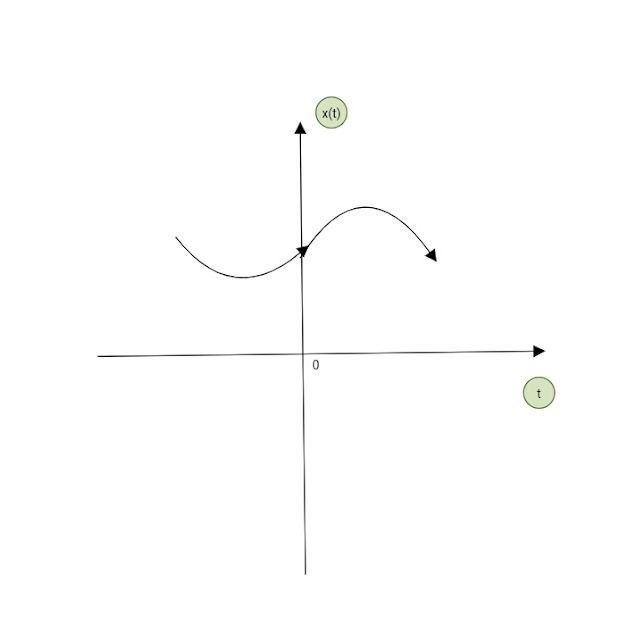### Ticker

6/recent/ticker-posts

# SIGNALS & CLASSIFICATION OF SIGNALS WITH FIGURE

Signals and Systems

Signals :-

A Signal is a single valued function of one or more variables that conveys some information. Signals are Represented mathematically as the functions of one or more independent variables.

A Signal may be a function of Time, Temperature, position, pressure, distance etc.
In Electrical sense, the Signal can be Voltage or current. The voltage or current is the function of Time as an independent variable.

Classification of Signals.
Signals may be classified in several ways:

❏ The signals can be one - dimensional or Multidimensional

❍ One dimensional Signals :- When the function depends on a Single variable, the signal is said to be one dimensional. Speech Signal, whose amplitude varies with time, is one - dimensional Signal.

❍ Multidimensional Signals :- Which the
function depends on two or more variables, the signal is said to be Multidimensional Signal. Image is an Multidimensional Signal because it is a two - dimensional Signal with horizontal and vertical coordinates.

❏ Based upon their mature and characteristics in the time domain, the signals my be broadly classified as given below:

❍ Continuous Time Signals :- The fuction is defined continuously in the time domain. A continuous - time Signal is Represented by x(t) where x Represents the shape of the signal and t shows that the variable is time.
This Signal will have some value at every instant of time.

Figure shows a continuous- time Signal.A Continuous - time Signal

❍ Discrete - Time Signals :- A discrete- time Signal is defined only at certain time - instant. For discrete- time Signals,
the amplitude between two time instant is not defined.A Discrete - time Signal

It is Represented by x(n) , here time n is the independent variable. Figure shows a discrete- time Signal.

Mathematically, a discrete- time Signal is denoted as under;

x(n) = { ...0, 0, 1, 3, 0, -2, 1, 2, 0...}
↑

Here arrow indicates the value of x(n) at
n = 0

❏ Deterministic and Non -Deterministic (Random) Signals

❍ Deterministic signals are those Signals which can be completely specified in time.
The pattern of this type of Signal is regular can be characterized mathematically.

Also the nature and amplitude of such a Signal at any time can be predicted.
So these Signals are called Deterministic Signals.

A Non - Deterministic Signal is one whose occurrence is always random in nature. The pattern of such a Signal is quite irregular so these Signal are also called random Signals.
For example, a thermal noise generated in an electric Circuit is a non - deterministic Signal.

❏  Periodic and Non - periodic
Signals :-

❍ Periodic Signals :- A Signal which repeat itself after a fixed time period is called a periodic Signal. It can be defined mathematically as

x(t) = x( t + T0 ). It is a condition of periodicity.

Here is T0 is called as the period of Signal x(t). Example of these Signals are sine wave, Cosine wave, square wave, etc.

❍ Periodic discrete - time Signal :- For the discrete - time Signal, the condition of periodicity is

x(n) = x( n+N )

Here, N is the period of Signal and its smallest value is called as fundamental period.

❍ Non - periodic Signal :- A Signal which does not repeat itself after a fixed Time Period or does not repeat at all is called as non - periodic or aperiodic Signal. Mathematical expression for this Signal is

x(t) ≠ x(t + T0)

Sometimes value of period T0 = ∞ then it is an exponential Signal and mathematically can be expressed as

x(t) = e^-αt

❍ Non - Periodic Discrete- time Signal :- Mathematical expression for this Signal is,

x(n) ≠ x (n + N)